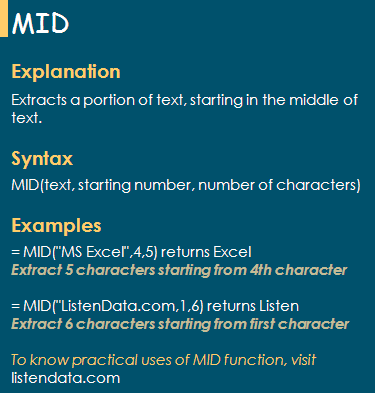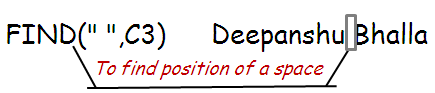# Excel MID Function and Its UsesExcel MID Function

Explanation

The MID function extracts a portion of text, starting in the middle of text.

Syntax

The syntax for MID function is as follows:
= MID( text , starting number , number of characters to extract)

Simple Uses of MID Function

1. To extract the first five characters from name.

Follow the steps shown in the image below.

2. To extract five characters from name, starting from the third character.

Follow the steps shown in the image below.

Practical Uses of MID Function

1. Extract First Name

Suppose a person's full name is entered in cell A2. Enter the following formula in cell B2

Logic : We need to extract characters till last character before space.

=MID (A2,1,FIND(" ",A2)-1)

1 => Start from first character

The FIND Function finds the position of a portion of text in a text.
In this case, it finds the position of a space in the name.2. Extract Last Name

Suppose a person's full name is entered in cell A2. Enter the following formula in cell B2

Logic : We need to extract characters starting from first character after space.

=MID (A2,FIND(" ",A2)+1,25)

The FIND Function finds the position of a space in the name.

25 : I assumed maximum number of characters in a last name would be 25.

3. Convert a Text Value into Date

=DATE(LEFT(A2,4),MID(A2,5,2),RIGHT(A2,2))

The DATE Function returns the sequential serial number that represents a particular date.

DATE(year,month,day)

LEFT(A2,4) => 2013

MID(A2,5,2) => 12

RIGHT(A2,2) => 09
Related Posts
Share1.